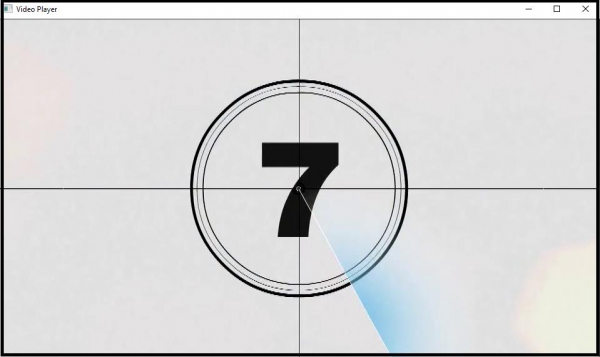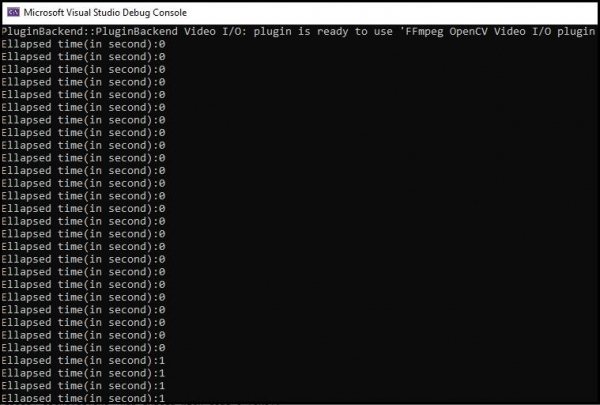# How to calculate Elapsed time in OpenCV using C++?

Here, we will understand how to calculate the elapsed time using OpenCV.

The following program calculates the elapsed time in OpenCV using C++.

## Example

#include<opencv2/opencv.hpp>//OpenCV header to use VideoCapture class//
#include<iostream>
using namespace std;
using namespace cv;
int main() {
Mat myImage;//Declaring a matrix to load the frames//
namedWindow("Video Player");//Declaring the video to show the video//
VideoCapture cap("video.mp4");//Declaring an object to load video from device//
if (!cap.isOpened()){ //This section prompt an error message if no video stream is found//
cout << "No video stream detected" << endl;
system("pause");
return-1;
}
while (true){ //Taking an everlasting loop to show the video//
cap >> myImage;
int elapsed_time;//Declaring an integer variable to store the elapsed time//
cout << "Ellapsed time(in second):" << elapsed_time / 1000 << endl;//Showing the elapsed time in seconds//
if (myImage.empty()){ //Breaking the loop if no video frame is detected//
break;
}
imshow("Video Player", myImage);//Showing the video//
char c = (char)waitKey(25);//Allowing 25 milliseconds frame processing time and initiating break condition//
if (c == 27){ //If 'Esc' is entered break the loop//
break;
}
}
cap.release();//Releasing the buffer memory//
return 0;
}

This program will play the video and will show elapsed time in the console window.

## Output